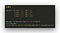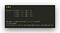# Sorting, finding max and min value from a list of dictionaries in python

In this article, we shall perform the following operations to a list of dictionaries.

1. sorting (ascending and descending)

Consider we have a list of dictionary like this,

`my_list = [{'name': 'A', 'age': 3},           {'name': 'B', 'age': 12},           {'name': 'C', 'age': 9},           {'name': 'D', 'age': 15}]`

We can use the following method to perform the above-mentioned operations on this list of dictionaries in a one-liner with the help of a lambda function.

# 1. Sorting

We can use the sorted() method to sort the list of dictionaries. The sort method takes the iterable as one of the arguments, a key argument where we can specify based on what the sorting should be, and then finally a reverse argument which takes a boolean value.

syntax

`sorted(list, key=condition, reverse=True)`

We can use the lambda function to get a specific key from the dictionary. The lambda function probably looks like this.

`lambda x: x['age']`

This will take an iterable and check for the value of ‘age’ in each of the iterable so that the sorting can be done based on that value.

The complete example looks like this.

sorting a list of dictionary in ascending order

The output of the above code is,

`[{'name': 'A', 'age': 3}, {'name': 'C', 'age': 9}, {'name': 'B', 'age': 12}, {'name': 'D', 'age': 15}]`

We can also sort this in reverse, by providing an extra argument called reverse which takes a boolean value. Specifying reverse=True will sort the list of dictionary in descending order.

sorting a list of dictionaries in descending order

The output of the reversed list is,

`[{'name': 'D', 'age': 15}, {'name': 'B', 'age': 12}, {'name': 'C', 'age': 9}, {'name': 'A', 'age': 3}]2.`

# 2. max

Finding the element with a maximum value based on a key-value can also be achieved in a one-liner using the max() method. The max method is also similar to the sort method in terms of the arguments passed to the function.

syntax

`max(iterable, key=lambda_function)`

The complete code snippet will look like this.getting the maximum object from a dictionary based on a key-value

getting the maximum object from a dictionary based on a key-value

The output of the above code is,

`{'name': 'D', 'age': 15}name D, age 15`

# 3. min

Similarly, we can also find the minimum value from a list of dictionaries based on a key-value. We will be using the min() method for this purpose. It takes the same arguments as the max() method.

syntax

`min(iterable, key=lambda_function)`

The complete code will look like this.getting the minimum object from a dictionary based on a key-value

getting the minimum object from a dictionary based on a key-value

The output of the above code is,

`{'name': 'A', 'age': 3}name A, age 3`

# Conclusion

These are the most efficient ways of performing sort, max, and min operations to a list of dictionaries. They are efficient and one-lined. There is no for loops are any such things. The task is achieved with the help of built-in methods only. Hope this article is helpful.

Python Developer | Django Developer | Python Enthusiast

## More from Allwin Raju

Python Developer | Django Developer | Python Enthusiast